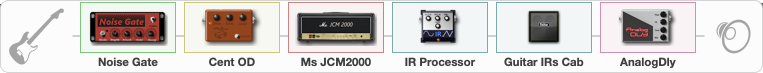# solo

Discussion in 'ToneLib-GFX presets' started by carlinos3, May 8, 2022.

1. solo

Preset name: carlos

Effects chain:Effect: "Noise Gate" (Dynamics / Filter), active - "yes"
{
"Mode" = Manual
"Depth" = 50
"Threshold" = 50
"Attack" = 0
"Hold" = 5
"Decay" = 250
}

Effect: "Cent OD" (Overdrive / Distortion), active - "yes"
{
"Drive" = 38
"Tone" = 77
"Level" = 60
}

Effect: "Ms JCM2000" (Amp simulators), active - "yes"
{
"Gain" = 100
"Bass" = 50
"Middle" = 62
"Treble" = 50
"Presence" = 55
"Master" = 100
"Level (dB)" = 0
}

Effect: "IR Processor" (Cabinets), active - "yes"
{
"IR" = charv.1_dc
"Low Cut (Hz)" = 0
"Hi Cut (kHz)" = 20.0
"Mix" = 100
"Level (dB)" = 5
}

Effect: "Guitar IRs Cab" (Cabinets), active - "yes"
{
"Model" = Marshall 1960A (4x12")
"Mic Position" = Middle
"Mic Distance" = Near
"Low Cut (Hz)" = 60
"Hi Cut (kHz)" = 20.0
"Mix" = 23
"Level (dB)" = 0
}

Effect: "AnalogDly" (Delay), active - "yes"
{
"Time" = 380
"Feedback" = 34
"Tone" = 65
"Mix" = 42
}

Note: You will need to download and install the ToneLib-GFX software to use the preset.

#### Attached Files:

• ###### solo.tlgfx
File size:
59 KB
Views:
2,101
Roman Nestor likes this.